Courses

# Test: Introduction To Heights And Distances

## 20 Questions MCQ Test Mathematics (Maths) Class 10 | Test: Introduction To Heights And Distances

Description
This mock test of Test: Introduction To Heights And Distances for Class 10 helps you for every Class 10 entrance exam. This contains 20 Multiple Choice Questions for Class 10 Test: Introduction To Heights And Distances (mcq) to study with solutions a complete question bank. The solved questions answers in this Test: Introduction To Heights And Distances quiz give you a good mix of easy questions and tough questions. Class 10 students definitely take this Test: Introduction To Heights And Distances exercise for a better result in the exam. You can find other Test: Introduction To Heights And Distances extra questions, long questions & short questions for Class 10 on EduRev as well by searching above.
QUESTION: 1

Solution:
QUESTION: 2

Solution:
QUESTION: 3

### From the given figure, find h.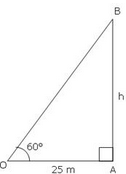​

Solution:
QUESTION: 4

The angle formed by the line of sight with the horizontal, when the point being viewed is above the horizontal level is called:​

Solution:

The term angle of elevation denotes the angle from the horizontal upward to an object.  An observer’s line of sight would be above the horizontal. And The term angle of depression denotes the angle from the horizontal downward to an object.  An observer’s line of sight would be below the horizontal. So The angle is called Angle of elevation.

QUESTION: 5

A tower stands vertically on the ground from a point on the ground which is 25 m away from the foot of tower if the height of tower is 25√3 metres find the angle of elevation.​

Solution:
QUESTION: 6

The angle of elevation of the sun, when the length of the shadow of a tree is equal to the height of the tree, is:

Solution: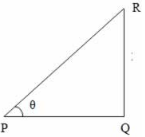Consider the diagram shown above where QR represents the tree and PQ represents its shadow

We have, QR = PQ
Let ∠QPR = θ
tan θ = QR/PQ = 1 (since QR = PQ)
⇒ θ = 45°

i,e., required angle of elevation = 45°

QUESTION: 7

If the length of a shadow cast by a pole is √3 times the length of the pole, then the angle of elevation of the sun is​

Solution:

Let AB be the tree and AC be its shadow.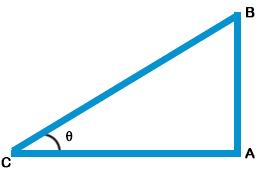Let ∠ACB = θ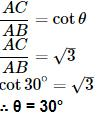QUESTION: 8

Let AC be the rope whose length is 20 m, and AB be the vertical pole of height h m and the angle of elevation of A at point C on the ground is 30°.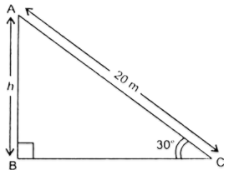Solution: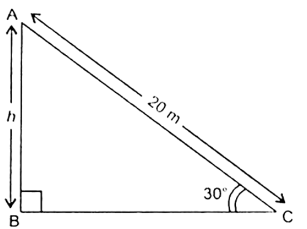Fif. 9.11
i.e., ∠ACB = 30°
In right triangle ABC, we have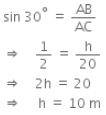Hence, the height of the pole is 10 m.

QUESTION: 9

A 20 m long ladder touches the wall at a height of 10 m. The angle which the ladder makes with the horizontal is

Solution:
QUESTION: 10

The ratio of the length of a rod and its shadow is 1:1/√3. what is the angle of elevation of the source of light

Solution:
QUESTION: 11

If sun’s elevation is 60° then a pole of height 6 m will cast a shadow of length

Solution:
QUESTION: 12

The angle of elevation from a point 30 metre from the base of tree as level ground to the top of the tree is 60°. The height of the tree is :

Solution:

Angle of elevation is 60
Base = 30m
Height of the tree = Perpendicular
So in the right triangle
Where base is given and we have to find perpendicular we have only tan θ
So, Tan θ = P/B
Tan 60 = P/30
√30 = P/30
P = 30√30

QUESTION: 13

Tree is broken by the wind the top struck the ground at 30degree at a distance of 30m. away from the root. Find the height of the tree.

Solution:

Let BD be the whole height of the tree

The tree breaks at point C and makes ΔABC as given into the figure such that

AC = CD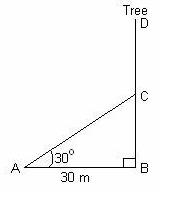In rt. ΔABC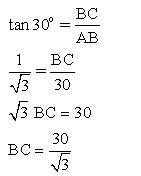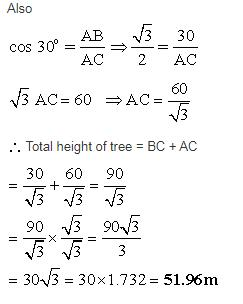QUESTION: 14

Find AB in the given figure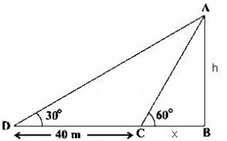Solution:

Use tanθ formula in both the triangles ABC and ABD.

QUESTION: 15

Guddi was standing on a road near a mall. She was 1000m away from the mall and able to see the top of the mall from the road in such a way that top of the tree, which is in between her and the mall, was exactly in line of sight with the top of the mall. The tree height is 10m and it is 20m away from Guddi. How tall is the mall?

Solution: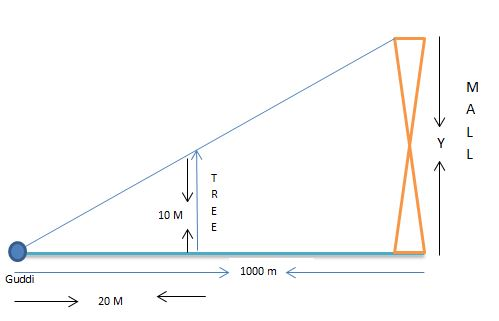Angle of elevation for Guddi is same for the tree and the mall.

So, the ratio of object height to its shadow will be same for the both subjects.

Therefore by proportionality rule,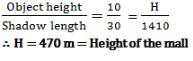QUESTION: 16

A tower stands vertically on the ground. From a point C on the ground, which is 20 m away from the foot of the tower, the angle of elevation of the top of the tower is found to be 450. The height of the tower is

Solution:

Angle of elevation =45 degrees
Base=20 m
Height of the tower=perpendicular
We have tan 45=P/B
1=P/20
P=20 m
Hence the height of the tower  is 20 metres.

QUESTION: 17

The angle of elevation from a point 30 feet from the base of a pole, of height h, as level ground to the top of the pole is 45o. Which equation can be used to find the height of the pole.

Solution:
QUESTION: 18

A tree casts a shadow 4 m long on the ground, when the angle of elevation of the sun is 45°. The height of the tree is:​

Solution:

In a triangle with one angle being 90 degrees (which the tree makes with the ground) and the other being 45 degrees (the angle of elevation), the 3rd angle is bound to be 45 degrees (180 - 90 - 45 = 45).

We also know that sides opposite to equal angles are equal.

Hence, the height of the tree will also be 4m.

QUESTION: 19

The angle of elevation of the sun when the length of the shadow of the tree is √3 times the height of the tree is​

Solution:
QUESTION: 20

A tower stands vertically on the ground. From a point on the ground which is 25 m away from the foot of the tower, the angle of elevation of the top of the tower is found to be 45o. Then the height (in meters) of the tower is​

Solution: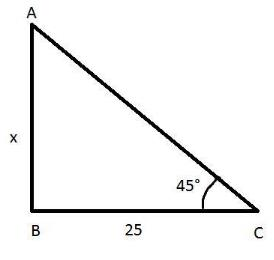A point on the ground which is 25 m away from the foot of the tower i. BC= 25 m

Let the height of the tower be x

The angle of elevation of the tower is found to be 45 degree.i.e.∠ACB=45°

In ΔABC

Using trigonometric ratios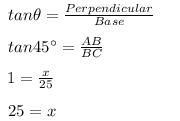Hence the height of the tower is 25 m.